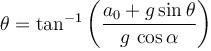Courses

# Newton's Law Of Motion MCQ Level - 2

## 10 Questions MCQ Test Topic wise Tests for IIT JAM Physics | Newton's Law Of Motion MCQ Level - 2

Description
This mock test of Newton's Law Of Motion MCQ Level - 2 for IIT JAM helps you for every IIT JAM entrance exam. This contains 10 Multiple Choice Questions for IIT JAM Newton's Law Of Motion MCQ Level - 2 (mcq) to study with solutions a complete question bank. The solved questions answers in this Newton's Law Of Motion MCQ Level - 2 quiz give you a good mix of easy questions and tough questions. IIT JAM students definitely take this Newton's Law Of Motion MCQ Level - 2 exercise for a better result in the exam. You can find other Newton's Law Of Motion MCQ Level - 2 extra questions, long questions & short questions for IIT JAM on EduRev as well by searching above.
QUESTION: 1

### A plank is held at an angle α to the horizontal (Figure) on two fixed supports A and B. The plank can slide against the supports (without friction) because of its weight Mg. Find the acceleration and direction in which a man of mass m should move so that the plank does not move.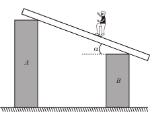Solution:

F.B.D. of man and plank are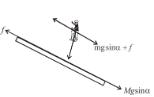For plank to be a rest, applying Newton's second law to plank along the incline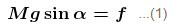and applying Newton's second law to man along the incline.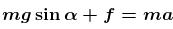........(2)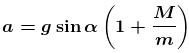down the incline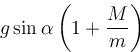down the incline

QUESTION: 2

### A particle of mass 2kg moves with an initial velocity of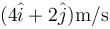on the xy-plane. A force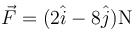acts on the particle. The initial position of the particle is (2m, 3m). Then for y = 3m,

Solution: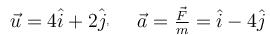Let at any time, the coordinates be (x,y).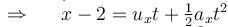and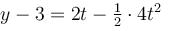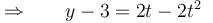When  y = 3m, t = 0, 1sec;      when t = 0, x = 2m;
when   t = 1sec,  x = 6.5m
The correct answer is: Possible value of is not only x = 2m, but there exist some other value of x also

QUESTION: 3

### Mass m shown in the figure is in equilibrium. If it is displaced further by x and released, find its acceleration just after it is released. Take pulleys to be light and smooth and string light.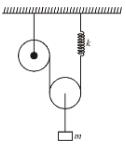Solution:

Initially the block is at rest under action of force 2T upward and mg downwards. When the block is pulled downwards by x, the spring extends by 2x. Hence tension T increases by 2kx. Thus the net unbalanced force on block of mass m is 4kx.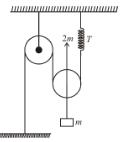∴ acceleration of the block is =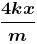The correct answer is: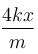QUESTION: 4

A block of mass m lies on wedge of mass M, which lies on fixed horizontal surface. The wedge is free to move on the horizontal force of magnitude F is applied on block as shown, neglecting friction at all surfaces, the value of force F such that block  has no relative motion w.r.t. wedge will be : (where g is acceleration due to gravity)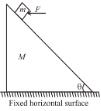Solution:

For no relative motion between wedge and block, let the acceleration of both block and wedge be a  towards left.
From FBD of block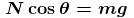...(1)
and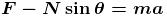......(2)
From FBD of wedge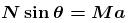...(3)
from equation (1), (2) and (3) solving we get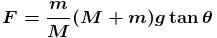The correct answer is: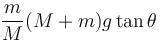QUESTION: 5

In the figure shown the acceleration of A is,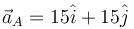then the acceleration of B is : (A remains in contact with B)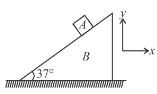Solution: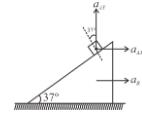From wedge constraint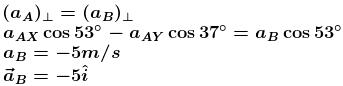The correct answer is: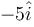QUESTION: 6

A cylinder rest in a supporting carriage as shown. The side AB of carriage makes an angle 30° with the horizontal and side BC is vertical. The carriage lies on a fixed horizontal surface and is being pulled towards left with an horizontal acceleration a. The magnitude of normal reactions exerted by sides AB and BC of carriage on the cylinder be NAB and NBC respectively. Neglect friction everywhere. Then as the magnitude of acceleration a of the carriage is increased, pick up the correct statement :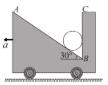Solution:

The force body diagram of cylinder is as shown.
Since net acceleration of cylinder is horizontal.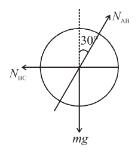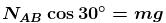or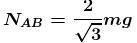......(1)
and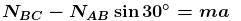or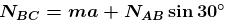...(2)
Hence NAB remains constant and NBC increases with increase in acceleration a.
The correct answer is: NAB remains constant and NBC increases

QUESTION: 7

A light spring is compressed and placed horizontally between a vertical fixed wall and a block free to slide over a smooth horizontal table top as shown in the figure. The system is released from rest. The graph which represents the relation between the magnitude of acceleration a of the block and the distance x traveled by it (as long as the spring is compressed) is :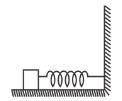Solution:

Let the initial compression of spring be l. Then the acceleration after the block travels a distance x is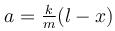⇒ ∴ The graph of a vs x is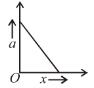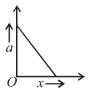QUESTION: 8

Four identical metal butterflies are hanging from a light string of length 5l at equally placed points as shown in the figure. The ends of the string are attached to a horizontal fixed support. The middle section of the string is horizontal. The relation between the angle  θ1 and θ2  is given by :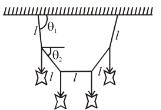Solution: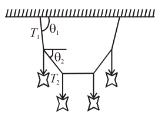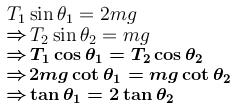The correct answer is: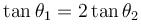QUESTION: 9

A block is lying on the horizontal frictionless surface. One end of a uniform rope is fixed to the block which is pulled in the horizontal direction by applying a force F at the other end. If the mass of the rope is half the mass of the block, the tension in the middle of the rope will be

Solution:

The acceleration of block-rope system is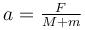where M is the mass of block and m is the mass of the rope.
So the tension in the middle of the rope will be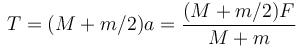Given that m = M/2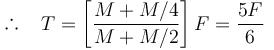QUESTION: 10

A pendulum of mass m hangs from a support fixed to a trolley. The direction of the string when the trolley rolls up a plane of inclination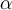with acceleration a0 is :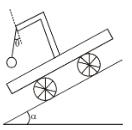Solution: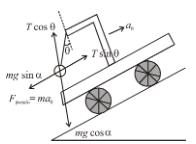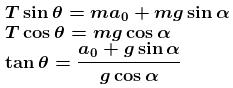The correct answer is: